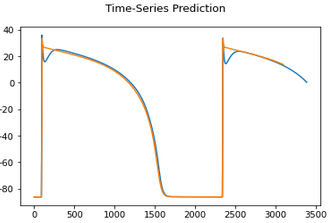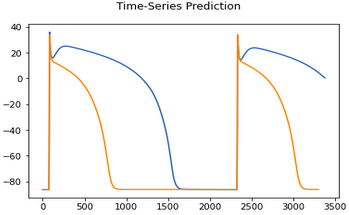# Prediction of Specific Section of Target Data in LSTM

Hello,
I am trying to obtain a wave model which requires simultaneous solutions of multiple ODE’s.
My input is has 2 input features. First one is Stimuli which only initiates the wave, and mostly 0’s.

However, my model can’t produce a specific part of the wave.As you can see, most of the wave is predicted very accurately.
What I tried is,

• Changing the hidden dimension
• Changins the sequence length
• Changing the batch size
• Increasing and decreasing LSTM Layer numbers
• Using MSE and L1 Loss functions
• Increasing data sizes

When I try to make my model more complex (such as increasing number of layers & size of hidden dimension) It tends to overfit,Exact opposite effect occurs when model is simplified or regularization is added.

How can it learn the area after initial sharp increase ? Is there a known approach for this ? I am at a loss at this point.

Thank you kindly.

Hey,
I am also applying LSTMs for circuit behavior modelation.
Did you pay attention to exploding and vanishing gradients?
Do you shuffle the data in the DataLoader?
Do you reduce the learning rate?

Hello Andre,
It sounds your problem is a regression problem as well ? This here is an electrophysiological wave model, we might be working on similar models.
I am using adaptive learning rate starting around e-5 with gamma = 0.1
I do use the shuffle = True for my training data loader. I guess that is what you meant?
I didn’t pay much attention to gradients since, my sequence lengths were relatively short and I was not sure on how to modify LSTM in that regard.

When I make the model even slightly more complex it starts making way shorter waves like in the first post which I assume due to overfitting.
I also have only 2 inpus namely stimuli and the electrical potential (which I am predicting).

It is an amplifier wave

1 Like